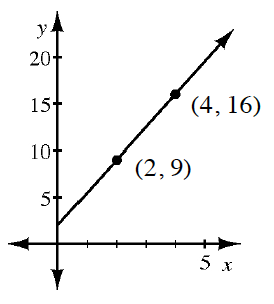### Home > INT1 > Chapter 3 > Lesson 3.1.1 > Problem3-13

3-13.

Draw a slope triangle and use it to write the equation of the line shown in the graph at right.

Review the Math Notes box in Lesson 2.2.2.

$y = \frac{7}{2}\textit{x}+2$